•5星
3.3MB weixin_44510615 2021-06-22 10:50:36
• 深度学习之路（四）matplotlib使用 Matplotlib 人工智能 python

matplotlob,是python里辅助制作图表的代码库，属于做机器学习的基础几个库之一。 Matplotlib是一个Python 2D绘图库，可以生成各种硬拷贝格式和跨平台的交互式环境的出版物质量数据。Matplotlib可用于Python脚本，...

matplotlib 介绍

matplotlob,是python里辅助制作图表的代码库，属于做机器学习的基础几个库之一。
Matplotlib是一个Python 2D绘图库，可以生成各种硬拷贝格式和跨平台的交互式环境的出版物质量数据。Matplotlib可用于Python脚本，Python和IPython shell，Jupyter笔记本，Web应用程序服务器和四个图形用户界面工具包。

Matplotlib试图让简单的事情变得简单而艰难。您只需几行代码即可生成绘图，直方图，功率谱，条形图，错误图，散点图等。有关示例，请参阅 示例图和缩略图库。

对于简单的绘图，pyplot模块提供了类似MATLAB的接口，特别是与IPython结合使用时。对于高级用户，您可以通过面向对象的界面或MATLAB用户熟悉的一组函数完全控制线型，字体属性，轴属性等

Matpotlib的基本使用

这是一张使用plot患处的2D的属性分布示意图，用来说明图表的常用属性以及显示的位置初级图形绘制

我们可以根据数据简单的绘制

import matplotlib
import matplotlib.pyplot as plt
import numpy as np
import pandas as pd
from IPython.display  import *
%matplotlib inline
x=np.arange(1.11)
y=2*x+5
plt.title("Matylotlib demo")
plt.xlabel("x")
plt.ylabel("y")
plt.plot(x,y)
plt.show()它默认是不显示中文的，如果我们要先显示中文，我们可以先下载SimHei.ttf
字体，然后进行操作：

zhfont1=matplotlib.font_manager.FontProperties(fname="SimHei.ttf")
plt.xlabel("x轴",fontproperties=zhfont1)

或者直接可以用本机上的字体，确认找到本机上适合中文字体，然后操作：

plt.rcParams['font.family']=['STFangsong']

自定义格式以及颜色缩写

如果我们要显示虚线图，

x=np.arange(1.11)
y=2*x+5
plt.title("Matylotlib demo")
plt.xlabel("x")
plt.ylabel("y")
plt.plot(x,y,"--r")  #显示虚线红色
plt.show()

作为线性图的替代，可以通过向 plot() 函数添加格式字符串来显示离散值。 可以使用以下格式化字符

字符描述
-实线样式（默认样式）
短横线
-.点划线
:虚线
.点标记
,像素标记
o圆标记
*星形标记

以下是matplotlib用到的颜色的缩写

字符颜色
‘b’蓝色
‘g’绿色
‘r’红色
‘c’青色
‘m’品红色
‘y’黄色
‘k’黑色
‘w’白色

绘制多个内容

subplot()能够在同个图中绘制多个内容

import numpy as np
import matplotlib.pyplot as plt
# 计算正弦和余弦曲线上的点的 x 和 y 坐标
x = np.arange(0,  3  * np.pi,  0.1)
y_sin = np.sin(x)
y_cos = np.cos(x)
# 建立 subplot 网格，高为 2，宽为 1
# 激活第一个 subplot
plt.subplot(2,  1,  1)
# 绘制第一个图像
plt.plot(x, y_sin)
plt.title('Sine')
# 将第二个 subplot 激活，并绘制第二个图像
plt.subplot(2,  1,  2)
plt.plot(x, y_cos)
plt.title('Cosine')
# 展示图像
plt.show()几种常见图表的操作

Histogram(柱状图)

plt.hist(x, bins= 10, range= None, normed= False, weights= None, cumulative= False, bottom= None, histtype= 'bar', align= 'mid', orientation= 'vertical', rwidth= None, log= False, color= None, label= None, stacked= False)

属性名描述
x指定绘制直方图的数据
bins条形条数
ranggex轴数据范围
normed是否将直方图频数换成频率
weights参数可为每一个数据点设置权重
cumulative是否需要计算累计频数或频率
bottom添加基准线，默认是0
orientation设置垂直水平方式
rwidth条形宽度百分比
color填充色

data=pd.DataFrame([90,70,45,58,68,72],index=["a","b","c","d","e","f"])
plt.hist(data,facecolor='r',histtype="bar")
plt.rcParams['font.family']=['SimHei.ttf']
plt.xlabel("学生")
plt.ylabel("成绩")
plt.show()


![绘制图形](http://lansus.coding.me/image/matplotlib_demo3.png)

## Scatter Plot（散点图）

plt.scatter(
x,
y,
s=None,
c=None,
marker=None,
cmap=None,
norm=None,
vmin=None,
vmax=None,
alpha=None,
linewidths=None,
verts=None,
edgecolors=None,
*,
data=None,
**kwargs,
)

下面是主要属性

|属性名|描述|
|:-:|:-:|
|x|散点图dx轴数据|
|y|散点图y轴数据|
|s|区域面积的大小|
|colors|散点图填充颜色|
|marker|显示标记，自定义散点的形状，例如marker=','散点会变成正方形，具体看参照[这里]https://matplotlib.org/api/markers_api.html?highlight=matplotlib%20markers#module-matplotlib.markers)|
|cmap|颜色地图|
|norm|是否转换成频率，只有当c是floa类型才可以使用|
|vmax,vmin|与norm配套使用，调整数据亮度|
|alpha|透明度|
|linewidths|调整散点边缘线宽度|
|edgecolors|边缘颜色设置|

使用代码如下:


size=50
x=np.random.rand(size)
y=np.random.rand(size)
area=np.pi*(135*x**2)
colors=np.random.rand(size)
plt.scatter(x,y,s=area,c=colors,alpha=0.5,marker="o",linewidths=2)


![绘制图形](http://lansus.coding.me/image/matplotlib_demo4.png)

## Pie Chart(饼状图)

plt.pie(
x,
explode=None,
labels=None,
colors=None,
autopct=None,
pctdistance=0.6,
labeldistance=1.1,
startangle=None,
counterclock=True,
wedgeprops=None,
textprops=None,
center=(0, 0),
frame=False,
rotatelabels=False,
*,
data=None,
)

x=[25,25,50]
explode=(0.01,0.01,0.01)
labels=['xioaming','xiaocheng','xiaoxiao']
plt.pie(x,labels=labels,explode=explode,autopct="%.1f%%")


![绘制图形](http://lansus.coding.me/image/matplotlib_demo5.png)

## 3D Plot（3D图）

import matplotlib as cm
from mpl_toolkits.mplot3d import Axes3D
X=np.arange(-5,5,0.25)
Y=np.arange(-5,5,0.25)
X,Y=np.meshgrid(X,Y)
R=np.sqrt(X**2+Y**2)
Z=np.sin(R)
fig=plt.figure()
ax=Axes3D(fig)
ax.plot_surface(X,Y,Z,rstride=1,cstride=1)
plt.show()
`

![绘制图形](http://lansus.coding.me/image/matplotlib_demo6.png)

展开全文lansus 2019-05-27 11:10:44
• 深度学习的实验中，图形的绘制和数据的可视化非常重要。Matplotlib是用于绘制图形的库，使用Matplotlib可以轻松地绘制图形和实现数据的可视化。 # 1.绘制简单图形 我们通常使用matplotlib的pyplot模块绘制图形。...

在深度学习的实验中，图形的绘制和数据的可视化非常重要。Matplotlib是用于绘制图形的库，使用Matplotlib可以轻松地绘制图形和实现数据的可视化。

# 1.绘制简单图形

我们通常使用matplotlib的pyplot模块绘制图形。下面是一个绘制sin函数曲线的例子。

import numpy as np
import matplotlib.pyplot as plt # 将matplotlib.pyplot作为plt导入

#生成数据
x = np.arange(0,6,0.1)  #以0.1为单位生成0到6的数据
y = np.sin(x)           #对x的各个元素，应用NumPy的sin函数生成y

#绘制图形
plt.plot(x,y)           #根据x,y的数值绘制图形
plt.show()              #显示图形

对应生成图像：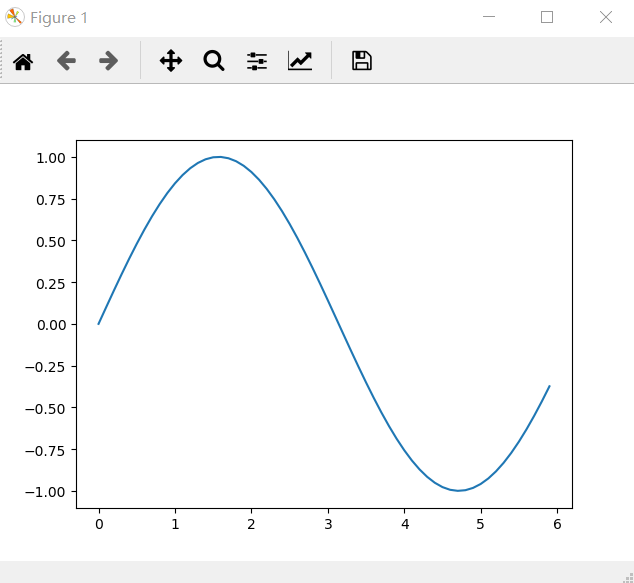# 2.pyplot的功能

下面的例子增加了cos函数图像的绘制，并使用了更多pyplot的功能。

import numpy as np
import matplotlib.pyplot as plt

# 生成数据
x = np.arange(0,6,0.1) # 生成0到6的数据，以0.1为单位
y1 = np.sin(x)         # 对数组x应用sin函数，得到数组y1
y2 = np.cos(x)         # 对数组x应用cos函数，得到数组y2

# 绘制图形
plt.plot(x,y1,label="sin")                 # 根据x和y1的取值绘制标签为sin的图形
plt.plot(x,y2,linestyle="--",label="cos")  # 根据x和y2的取值绘制标签为cos的图形，用虚线绘制

plt.xlabel("x")   # 设置x轴标签为"x"
plt.ylabel("y")   # 设置y轴标签为"y"

plt.title('sin&cos') # 设置标题为"sin&cos"

plt.legend() # 绘制图例

plt.show()   # 显示所绘制的图像

对应生成图像：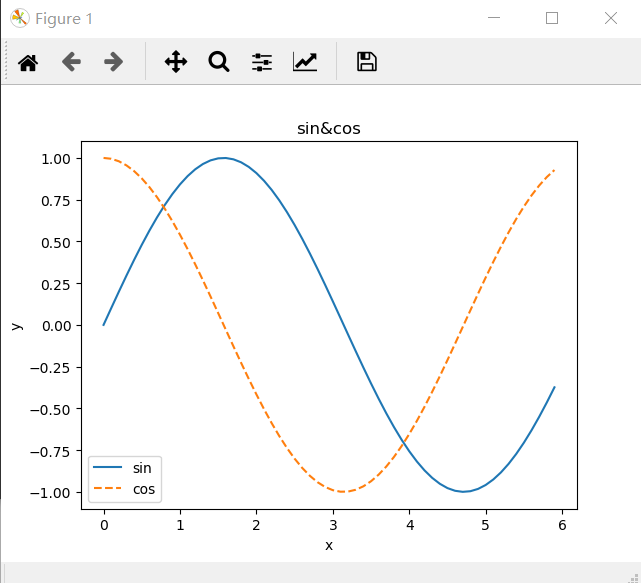# 3.显示图像

import matplotlib.pyplot as plt

plt.imshow(img)              #绘制图像

plt.show()                   #显示图像

对应显示图像效果：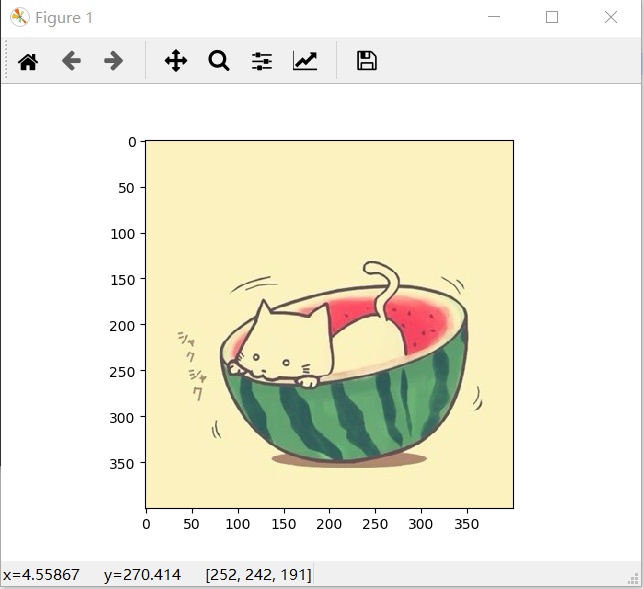# 本博客参考了《深度学习入门——基于Python的理论与实现》（斋藤康毅著，陆宇杰译），特在此声明。

展开全文weixin_44711653 2020-01-26 12:25:40
• 深度学习基础之matplotlib，一文搞定各个示例，建议收藏以后参考 深度学习 python 人工智能

汇总matplotlib的所有demo，收藏以后参考

目录

1、先来个demo 分析下

2、matplotlib中的概念

3、matplotlib 支持的图形

3.1 线图line

3.2 直方图hist

3.3 路线

3.4 散点图Scatter

3.5 极坐标图Polar plots

3.6 饼图pie

3.7 3d 图形

总结：

Matplotlib 是 Python 的绘图库。 它可与 NumPy 一起使用 ，Matplotlib也是深度学习的常用绘图库，主要是将训练的成果进行图形化，因为这样更直观，更方便发现训练中的问题，今天来学习下，走起！！

1、先来个demo 分析下

下面是一个典型的图形，我们如果想华这样一个图，应该做什么，应该准备哪些数据，这样的图形有哪些属性是你需要关注的？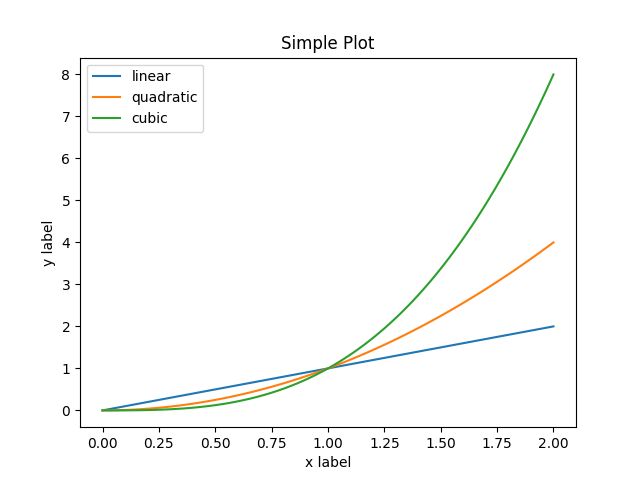1、怎么做一个图？ 怎么创建一个画板？

2、怎么设置数据？x，y 的数据怎么放上去

3、设置图标示，左上角的各种线的颜色这种小窗体怎么画上去？

4、外观 ，不同线的颜色不同怎么处理？

5、怎么显示？

6、怎么保存？

这么多的问题，怎么搞？一步一步来，先给你看看代码，看下能明白多少

x = np.linspace(0, 2, 100)
​
plt.plot(x, x, label='linear')  # Plot some data on the (implicit) axes.
plt.plot(x, x**3, label='cubic')
plt.xlabel('x label')
plt.ylabel('y label')
plt.title("Simple Plot")
plt.legend()

2、matplotlib中的概念

下面这张图是官网的一张图，指明了很多概念，基本上常用的我们都能看到，看起来大部分也都能理解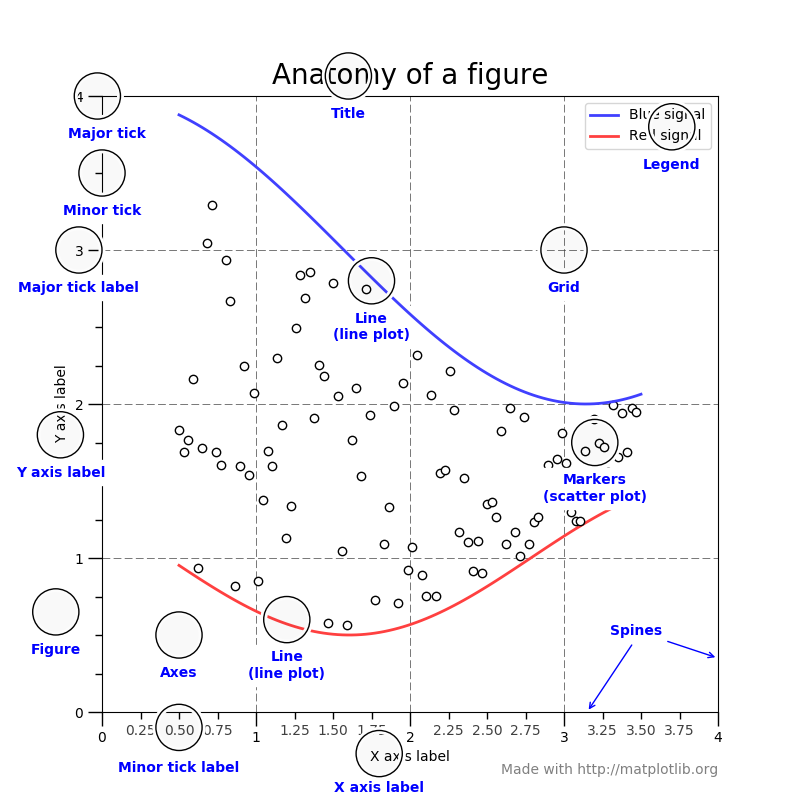Figure(容器)

整个图像称为Figure， Figure用于保存返回的Axes(坐标域)， 一个Figure可以包含任意数量的Axes，可以理解为一个容器。

Axes(坐标域)

可以将它理解为一个单个画板， 一个Axes包含两个Axis(坐标轴)(在3D图中为三个Axis), 每个Axes都有 一个 title(方法: set_title()), 一个x-label(方法: set_xlabel()), 一个y-label(方法: set_ylabel()).注意：一个给出的Axes对象只能包含在一个Figure中。

Axis(坐标轴)

这是一种类似数轴的对象。可以通过Axis以及Axis的方法设置坐标轴上刻度的样式和坐标轴上的值。刻度的位置由Locater对象决定， 刻度对应的值由Formatter对象决定。

总结：Axes 差不多就是我们脑中所想的一个 'plot'。可以说就是单张纸。一个确定的figure当中可以有很多个Axes，但是一个确定的Axes只能在一个figure当中。

2维空间的Axes包含两个Axis（即x轴与y轴），3维空间的Axes包含三个Axis（即x轴，y轴和z轴）。这里注意区别Axes和Axis的概念

Axis是一个数轴对象，它主要用于设置一个Axes里面的数据约束（即两个端点的值）和轴上的ticks（就是轴上的标记刻度）和tick-labels刻度的标签。

Subplot：子图，figure对象下创建一个或多个subplot对象(即axes)用于绘制图像。

axes: 设置坐标轴边界和表面的颜色、坐标刻度值大小和网格的显示

figure: 控制dpi、边界颜色、图形大小、和子区( subplot)设置

font: 字体集（font family）、字体大小和样式设置

grid: 设置网格颜色和线性

legend: 设置图例和其中的文本的显示

line: 设置线条（颜色、线型、宽度等）和标记

patch: 是填充2D空间的图形对象，如多边形和圆。控制线宽、颜色和抗锯齿设置等。

savefig: 可以对保存的图形进行单独设置。例如，设置渲染的文件的背景为白色。

xticks和yticks: 为x,y轴的主刻度和次刻度设置颜色、大小、方向，以及标签大小。

3、matplotlib 支持的图形

3.1 线图line

import matplotlib.pyplot as plt
import numpy as np
​
# Data for plotting
t = np.arange(0.0, 2.0, 0.01)
s = 1 + np.sin(2 * np.pi * t)
​
fig, ax = plt.subplots()
ax.plot(t, s)
​
ax.set(xlabel='time (s)', ylabel='voltage (mV)',
title='About as simple as it gets, folks')
ax.grid()
​
fig.savefig("test.png")
plt.show()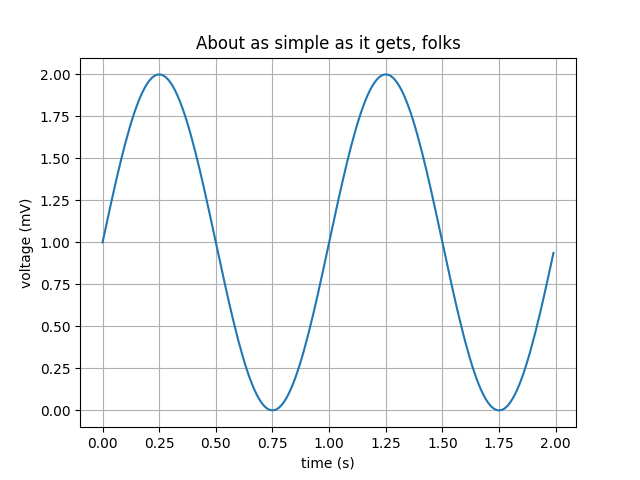3.2 直方图hist

import numpy as np
import matplotlib.pyplot as plt
​
np.random.seed(19680801)
​
# example data
mu = 100  # mean of distribution
sigma = 15  # standard deviation of distribution
x = mu + sigma * np.random.randn(437)
​
num_bins = 50
​
fig, ax = plt.subplots()
​
# the histogram of the data
n, bins, patches = ax.hist(x, num_bins, density=True)
​
# add a 'best fit' line
y = ((1 / (np.sqrt(2 * np.pi) * sigma)) *
np.exp(-0.5 * (1 / sigma * (bins - mu))**2))
ax.plot(bins, y, '--')
ax.set_xlabel('Smarts')
ax.set_ylabel('Probability density')
ax.set_title(r'Histogram of IQ: $\mu=100$, $\sigma=15$')
​
# Tweak spacing to prevent clipping of ylabel
fig.tight_layout()
plt.show()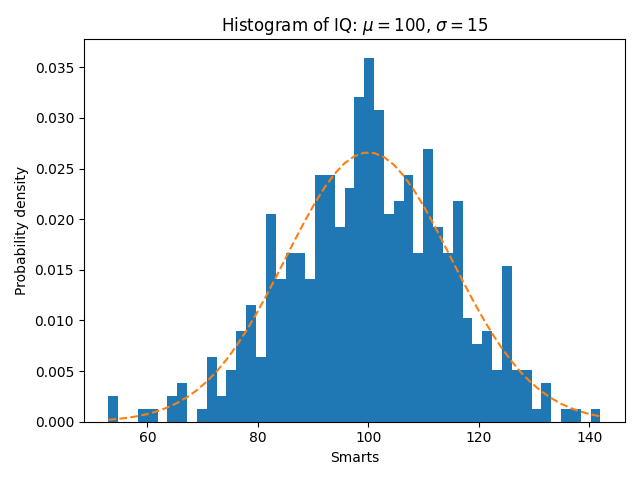3.3 路线

import matplotlib.path as mpath
import matplotlib.patches as mpatches
import matplotlib.pyplot as plt
​
fig, ax = plt.subplots()
​
Path = mpath.Path
path_data = [
(Path.MOVETO, (1.58, -2.57)),
(Path.CURVE4, (0.35, -1.1)),
(Path.CURVE4, (-1.75, 2.0)),
(Path.CURVE4, (0.375, 2.0)),
(Path.LINETO, (0.85, 1.15)),
(Path.CURVE4, (2.2, 3.2)),
(Path.CURVE4, (3, 0.05)),
(Path.CURVE4, (2.0, -0.5)),
(Path.CLOSEPOLY, (1.58, -2.57)),
]
codes, verts = zip(*path_data)
path = mpath.Path(verts, codes)
patch = mpatches.PathPatch(path, facecolor='r', alpha=0.5)
​
# plot control points and connecting lines
x, y = zip(*path.vertices)
line, = ax.plot(x, y, 'go-')
​
ax.grid()
ax.axis('equal')
plt.show()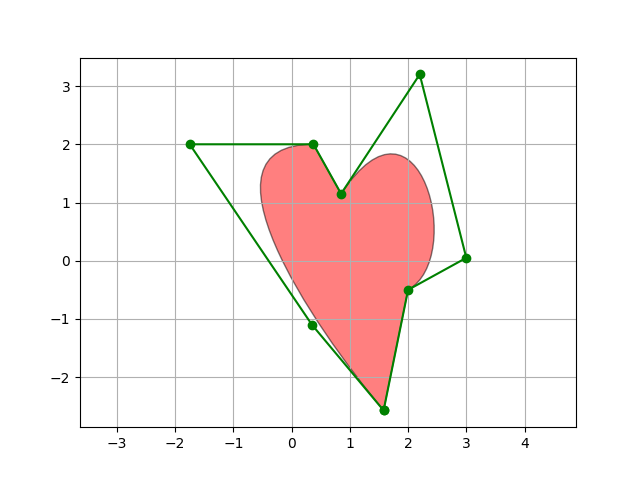3.4 散点图Scatter

import numpy as np
import matplotlib.pyplot as plt
import matplotlib.cbook as cbook

# Load a numpy record array from yahoo csv data with fields date, open, close,
# volume, adj_close from the mpl-data/example directory. The record array
# stores the date as an np.datetime64 with a day unit ('D') in the date column.
.view(np.recarray))
price_data = price_data[-250:]  # get the most recent 250 trading days

# Marker size in units of points^2
volume = (15 * price_data.volume[:-2] / price_data.volume)**2
close = 0.003 * price_data.close[:-2] / 0.003 * price_data.open[:-2]

fig, ax = plt.subplots()
ax.scatter(delta1[:-1], delta1[1:], c=close, s=volume, alpha=0.5)

ax.set_xlabel(r'$\Delta_i$', fontsize=15)
ax.set_ylabel(r'$\Delta_{i+1}$', fontsize=15)
ax.set_title('Volume and percent change')

ax.grid(True)
fig.tight_layout()

plt.show()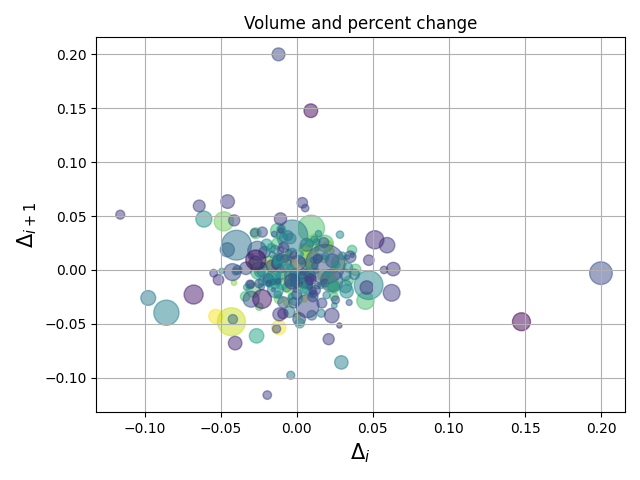3.5 极坐标图Polar plots

import numpy as np
import matplotlib.pyplot as plt
​
​
r = np.arange(0, 2, 0.01)
theta = 2 * np.pi * r
​
fig, ax = plt.subplots(subplot_kw={'projection': 'polar'})
ax.plot(theta, r)
ax.set_rmax(2)
ax.set_rticks([0.5, 1, 1.5, 2])  # Less radial ticks
ax.set_rlabel_position(-22.5)  # Move radial labels away from plotted line
ax.grid(True)
​
ax.set_title("A line plot on a polar axis", va='bottom')
plt.show()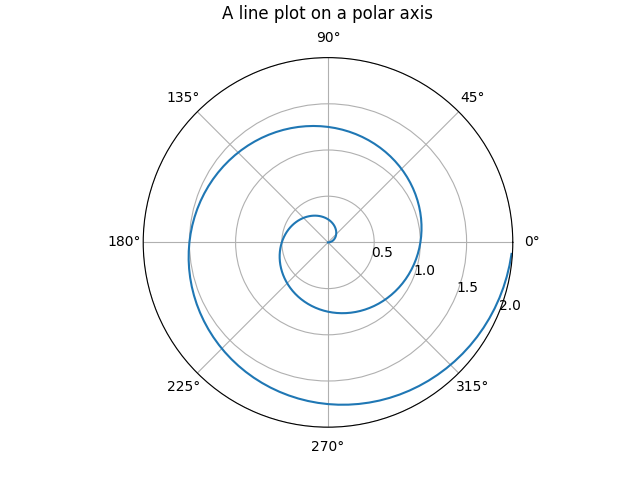3.6 饼图pie

import matplotlib.pyplot as plt
​
# Pie chart, where the slices will be ordered and plotted counter-clockwise:
labels = 'Frogs', 'Hogs', 'Dogs', 'Logs'
sizes = [15, 30, 45, 10]
explode = (0, 0.1, 0, 0)  # only "explode" the 2nd slice (i.e. 'Hogs')
​
fig1, ax1 = plt.subplots()
ax1.pie(sizes, explode=explode, labels=labels, autopct='%1.1f%%',
ax1.axis('equal')  # Equal aspect ratio ensures that pie is drawn as a circle.
​
plt.show()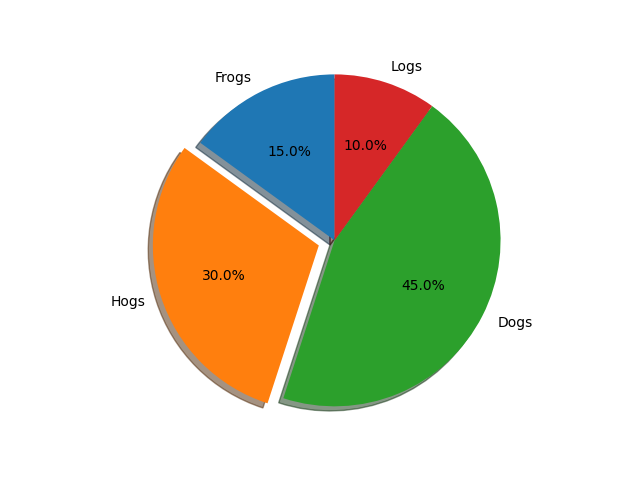3.7 3d 图形

import matplotlib.pyplot as plt
from matplotlib import cm
from matplotlib.ticker import LinearLocator
import numpy as np
​
fig, ax = plt.subplots(subplot_kw={"projection": "3d"})
​
# Make data.
X = np.arange(-5, 5, 0.25)
Y = np.arange(-5, 5, 0.25)
X, Y = np.meshgrid(X, Y)
R = np.sqrt(X**2 + Y**2)
Z = np.sin(R)
​
# Plot the surface.
surf = ax.plot_surface(X, Y, Z, cmap=cm.coolwarm,
linewidth=0, antialiased=False)
​
# Customize the z axis.
ax.set_zlim(-1.01, 1.01)
ax.zaxis.set_major_locator(LinearLocator(10))
# A StrMethodFormatter is used automatically
ax.zaxis.set_major_formatter('{x:.02f}')
​
# Add a color bar which maps values to colors.
fig.colorbar(surf, shrink=0.5, aspect=5)
​
plt.show()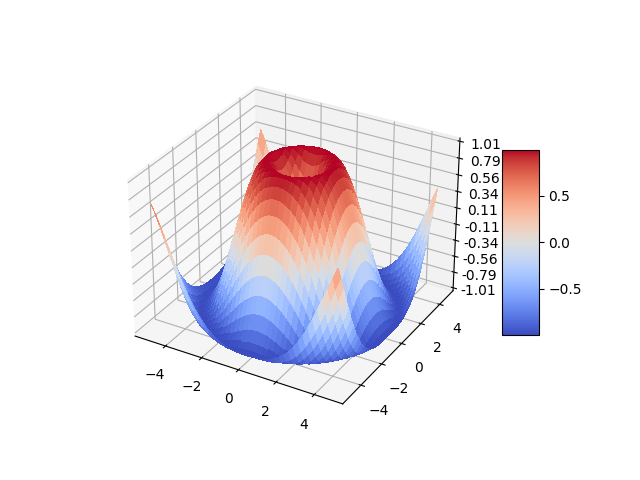#使用numpy产生数据
x=np.arange(-5,5,0.1)
y=x*3
​
#创建窗口、子图
#方法1：先创建窗口，再创建子图。（一定绘制）
fig = plt.figure(num=1, figsize=(15, 8),dpi=80)     #开启一个窗口，同时设置大小，分辨率
print(fig,ax1,ax2)
#方法2：一次性创建窗口和多个子图。（空白不绘制）
fig,axarr = plt.subplots(4,1)  #开一个新窗口，并添加4个子图，返回子图数组
ax1 = axarr    #通过子图数组获取一个子图
print(fig,ax1)
#方法3：一次性创建窗口和一个子图。（空白不绘制）
ax1 = plt.subplot(1,1,1,facecolor='white')      #开一个新窗口，创建1个子图。facecolor设置背景颜色
print(ax1)
#获取对窗口的引用，适用于上面三种方法
# fig = plt.gcf()   #获得当前figure
# fig=ax1.figure   #获得指定子图所属窗口
​
​
#设置子图的基本元素
ax1.set_title('python-drawing')            #设置图体，plt.title
ax1.set_xlabel('x-name')                    #设置x轴名称,plt.xlabel
ax1.set_ylabel('y-name')                    #设置y轴名称,plt.ylabel
plt.axis([-6,6,-10,10])                  #设置横纵坐标轴范围，这个在子图中被分解为下面两个函数
ax1.set_xlim(-5,5)                           #设置横轴范围，会覆盖上面的横坐标,plt.xlim
ax1.set_ylim(-10,10)                         #设置纵轴范围，会覆盖上面的纵坐标,plt.ylim
​
xmajorLocator = MultipleLocator(2)   #定义横向主刻度标签的刻度差为2的倍数。就是隔几个刻度才显示一个标签文本
ymajorLocator = MultipleLocator(3)   #定义纵向主刻度标签的刻度差为3的倍数。就是隔几个刻度才显示一个标签文本
​
ax1.xaxis.set_major_locator(xmajorLocator) #x轴 应用定义的横向主刻度格式。如果不应用将采用默认刻度格式
ax1.yaxis.set_major_locator(ymajorLocator) #y轴 应用定义的纵向主刻度格式。如果不应用将采用默认刻度格式
​
ax1.xaxis.grid(True, which='major')      #x坐标轴的网格使用定义的主刻度格式
ax1.yaxis.grid(True, which='major')      #x坐标轴的网格使用定义的主刻度格式
​
ax1.set_xticks([])     #去除坐标轴刻度
ax1.set_xticks((-5,-3,-1,1,3,5))  #设置坐标轴刻度
ax1.set_xticklabels(labels=['x1','x2','x3','x4','x5'],rotation=-30,fontsize='small')  #设置刻度的显示文本，rotation旋转角度，fontsize字体大小
​
plot1=ax1.plot(x,y,marker='o',color='g',label='legend1')   #点图：marker图标
plot2=ax1.plot(x,y,linestyle='--',alpha=0.5,color='r',label='legend2')   #线图：linestyle线性，alpha透明度，color颜色，label图例文本
​
ax1.legend(loc='upper left')            #显示图例,plt.legend()
ax1.text(2.8, 7, r'y=3*x')                #指定位置显示文字,plt.text()
ax1.annotate('important point', xy=(2, 6), xytext=(3, 1.5),  #添加标注，参数：注释文本、指向点、文字位置、箭头属性
arrowprops=dict(facecolor='black', shrink=0.05),
)
#显示网格。which参数的值为major(只绘制大刻度)、minor(只绘制小刻度)、both，默认值为major。axis为'x','y','both'
ax1.grid(b=True,which='major',axis='both',alpha= 0.5,color='skyblue',linestyle='--',linewidth=2)
​
axes1 = plt.axes([.2, .3, .1, .1], facecolor='y')       #在当前窗口添加一个子图，rect=[左, 下, 宽, 高]，是使用的绝对布局，不和以存在窗口挤占空间
axes1.plot(x,y)  #在子图上画图
plt.savefig('aa.jpg',dpi=400,bbox_inches='tight')   #savefig保存图片，dpi分辨率，bbox_inches子图周边白色空间的大小
plt.show()    #打开窗口，对于方法1创建在窗口一定绘制，对于方法2方法3创建的窗口，若坐标系全部空白，则不绘制

总结：

上面大部分的数据是总结自官方网站，

https://matplotlib.org/stable/tutorials/introductory/usage.html#sphx-glr-tutorials-introductory-usage-py

如果上面有说的不准确的可以参考官方网站，还不能完全掌握，我也只能画画简单的图，能满足日常的需求罢了

整理不容易，求点赞支持

展开全文perfect2011 2021-09-07 15:46:59
• 深度学习之Python的可视化包 – Matplotlib 深度学习 人工智能 机器学习 python

5.4 Python的可视化包 – Matplotlib Matplotlib是Python中最常用的可视化工具之一，可以非常方便地创建海量类型地2D图表和一些基本的3D图表。Matplotlib最早是为了可视化癫痫病人的脑皮层电图相关的信号而研发，...

5.4 Python的可视化包 – Matplotlib

Matplotlib是Python中最常用的可视化工具之一，可以非常方便地创建海量类型地2D图表和一些基本的3D图表。Matplotlib最早是为了可视化癫痫病人的脑皮层电图相关的信号而研发，因为在函数的设计上参考了MATLAB，所以叫做Matplotlib。Matplotlib首次发表于2007年，在开源和社区的推动下，现在在基于Python的各个科学计算领域都得到了广泛应用。Matplotlib的原作者John D. Hunter博士是一名神经生物学家，2012年不幸因癌症去世，感谢他创建了这样一个伟大的库。

安装Matplotlib的方式和numpy很像，可以直接通过Unix/Linux的软件管理工具，比如Ubuntu 16.04 LTS下，输入：

>> sudo apt install python-matplotlib

或者通过pip安装：

>> pip install matplotlib

Windows下也可以通过pip，或是到官网下载：

Matplotlib非常强大，不过在深度学习中常用的其实只有很基础的一些功能，这节主要介绍2D图表，3D图表和图像显示。

5.4.1 2D图表

Matplotlib中最基础的模块是pyplot。先从最简单的点图和线图开始，比如我们有一组数据，还有一个拟合模型，通过下面的代码图来可视化：

matplotlib和pyplot的惯用别名分别是mpl和plt，上面代码生成的图像如下：基本的画图方法就是这么简单，如果想了解更多pyplot的属性和方法来画出风格多样的图像，可以参考官网：

pyplot – Matplotlib 1.5.3 documentation

Customizing matplotlib

点和线图表只是最基本的用法，有的时候我们获取了分组数据要做对比，柱状或饼状类型的图或许更合适：5.3.1 3D图表

Matplotlib中也能支持一些基础的3D图表，比如曲面图，散点图和柱状图。这些3D图表需要使用mpl_toolkits模块，先来看一个简单的曲面图的例子：

这个例子中先生成一个所有值均为0的复数array作为初始频谱，然后把频谱中央部分用随机生成，但同时共轭关于中心对称的子矩阵进行填充。这相当于只有低频成分的一个随机频谱。最后进行反傅里叶变换就得到一个随机波动的曲面，图像如下：3D的散点图也是常常用来查看空间样本分布的一种手段，并且画起来比表面图和网线图更加简单，来看例子：

这个例子中，为了方便，直接先采样了一堆3维的正态分布样本，保证方向上的均匀性。然后归一化，让每个样本到原点的距离为1，相当于得到了一个均匀分布在球面上的样本。再接着把每个样本都乘上一个均匀分布随机数的开3次方，这样就得到了在球体内均匀分布的样本，最后根据判别平面3x+2y-z-1=0对平面两侧样本用不同的形状和颜色画出，图像如下：5.3.1 图像显示

Matplotlib也支持图像的存取和显示，并且和OpenCV一类的接口比起来，对于一般的二维矩阵的可视化要方便很多，来看例子：

这段代码中第一个例子是读取一个本地图片并显示，第二个例子中直接把上小节中反傅里叶变换生成的矩阵作为图像拿过来，原图和经过乘以3再加4变换的图直接绘制了两个形状一样，但是值的范围不一样的图案。显示的时候imshow会自动进行归一化，把最亮的值显示为纯白，最暗的值显示为纯黑。这是一种非常方便的设定，尤其是查看深度学习中某个卷积层的响应图时。得到图像如下：只讲到了最基本和常用的图表及最简单的例子，更多有趣精美的例子可以在Matplotlib的官网找到：

展开全文z4909801 2017-10-28 14:22:58
• 195KB weixin_38688403 2021-01-20 04:16:46
• 42KB weixin_42676876 2021-10-03 11:40:19
• Python - 深度学习训练过程使用matplotlib.pyplot实时动态显示loss和acc曲线 深度学习 matplotlib loss曲线 acc曲线

HW140701 2021-07-19 13:55:49
• 深度学习入门03-Matplotlib matplotlib python 深度学习入门

iMotherBoard 2019-07-18 21:28:06
• 深度学习pytorch入门之Matplotlib初步 python 深度学习 matplotlib

ZP_icenow 2019-11-24 18:11:18
• 深度学习框架 tensorflow PyTorch(torch) numpy matplotlib 安装 tensorflow pip python 深度学习 神经网络

JavaBigData 2020-08-15 13:38:28
• 深度学习一行代码使用matplotlib画出特征彩色图的color map 深度学习 计算机视觉 pytorch

slamdunkofkd 2020-03-26 10:58:57
• 学习神经网络&深度学习 Tensorflow2.0 Matplotlib绘图基础&学习日记1 数据可视化 python

sheldonkai 2020-03-04 12:02:03
• 50个令人大开眼界的 Matplotlib 可视化项目 可视化 数据可视化 人工智能 https python

weixin_47196664 2020-09-12 11:50:00
• Pytorch入门深度学习（5）——matplotlib的使用 python 深度学习 pytorch

wa1tzy 2020-06-02 20:48:49
• PyTorch深度学习——PyCharm中使用matplotlib画3D曲面图 pycharm 深度学习 python matplotlib

Learning_AI 2021-09-26 13:05:03
• 183KB weixin_38560107 2020-12-21 07:55:36
• weixin_43582101 2019-03-08 12:26:03
• 使用matplotlib显示图片（《深度学习入门：基于Python的理论与实现》实践笔记） python matplotlib

xswl5148 2021-07-17 13:44:39
• 深度学习原理详解及Python代码实现 人工智能 深度学习 python 卷积神经网络 循环神经网络

32.65MB weixin_27066963 2021-06-16 22:40:01
• vscode配置python、Anaconda、创建深度学习环境安装tensorflow、numpy、matplotlib（Windows） python anaconda

qq_22305897 2020-05-01 20:47:33
• 深度学习PIL以及Matplotlib基础知识 人工智能

weixin_44407811 2021-09-06 15:08:01
• 257KB weixin_38604330 2021-01-06 18:42:38
• 89KB weixin_38608693 2021-01-20 03:19:08
• liumiaocn 2018-06-24 10:48:57
• 85.9MB weixin_42099815 2021-02-03 08:37:07
• jorg_zhao 2018-10-31 13:31:58
• 27KB weixin_38747444 2021-01-06 19:20:29
• 深度学习Tensorflow,numpy,matplotlib,pandas,keras等清华镜像源下载指令 tensorflow 深度学习 神经网络

weixin_44824894 2020-09-11 00:21:49
• 深度学习入门笔记（十二）：深度学习数据读取 深度学习 数据读取 minibatch tfrecords h5py

TeFuirnever 2019-10-11 18:57:47...